# Texas Go Math Kindergarten Lesson 7.2 Answer Key Count and Write 11 and 12

Refer to our Texas Go Math Kindergarten Answer Key Pdf to score good marks in the exams. Test yourself by practicing the problems from Texas Go Math Kindergarten Lesson 7.2 Answer Key Count and Write 11 and 12.

## Texas Go Math Kindergarten Lesson 7.2 Answer Key Count and Write 11 and 12

Explore

DIRECTIONS: Count and tell how many. Trace and write the numbers and the words.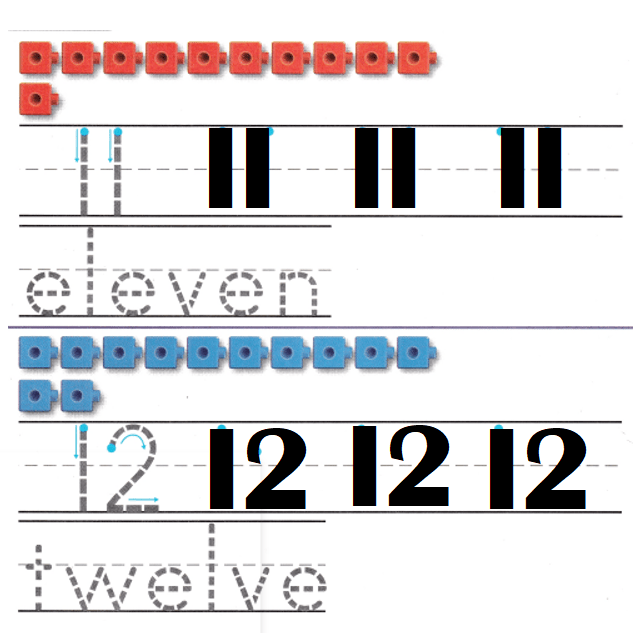Explanation:
Number of cubes = 11 or Eleven.
Number of cubes = 12 or Twelve.

Share and Show

DIRECTIONS: 1. Count and tell how many. Practice writing the number. 2. Count and tell how many. Write the number. 3. Look at the squares in Exercise 2. Write how many blue squares. Write how many red squares. Write how many squares in all.

Question 1.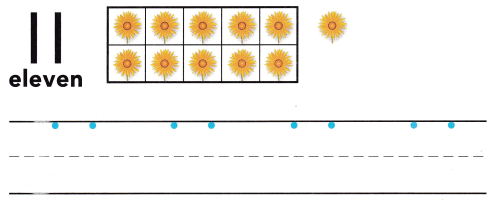Explanation:
Number of flowers = 11 or Eleven.

Question 2.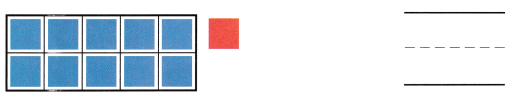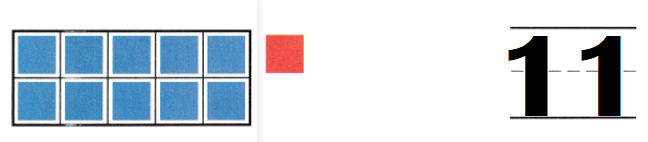Explanation:
Number of cubes = 10 + 1 = 11.

Question 3.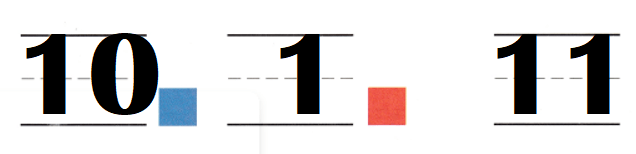Explanation:
Number of blue cubes = 10.
Number of blue cubes = 1.
Total number of cubes = Number of blue cubes + Number of red cubes
= 10 + 1
= 11.

DIRECTIONS: 4. Count and tell how many. Practice writing the number. 5. Count and tell how many. Write the number. 6. Look at the squares in Exercise 5. Write how many green squares. Write how many orange squares. Write how many squares in oil.

Question 4.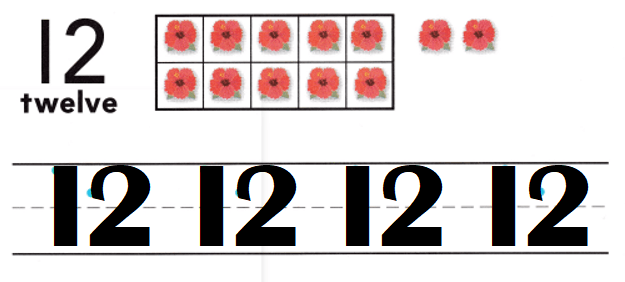Explanation:
Number of flowers = 12 or Twelve.

Question 5.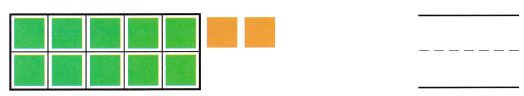Explanation:
Number of cubes = 12 or Twelve.

Question 6.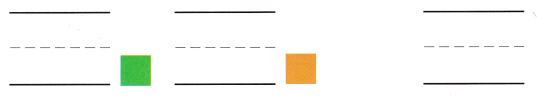Explanation:
Number of green cubes = 10.
Number of yellow cubes = 10.
Total number of cubes = Number of green cubes + Number of yellow cubes
= 10 + 2
= 12 or Twelve.

HOME ACTIVITY • Ask your child to count and write the number for a set of 11 or 12 objects, such as macaroni pieces or buttons.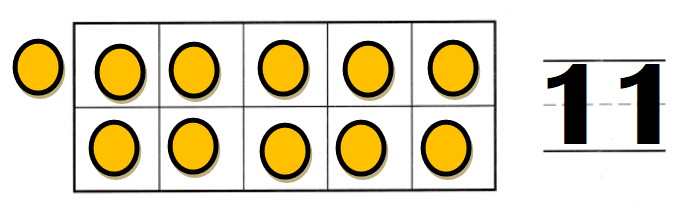Explanation:
My kid took buttons and counted them = 11 or Eleven.

DIRECTIONS: 7-8. Look at the number. Draw more flowers to show that number. 9. Count the counters. Write the number.

Problem Solving
Question 7.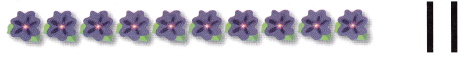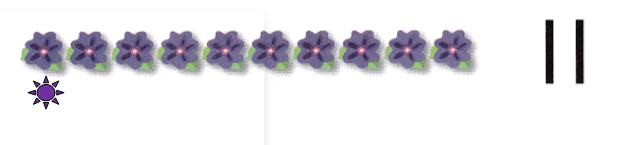Explanation:
Number of flowers = 10 + 1 = 11.

Question 8.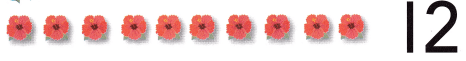Explanation:
Number of flowers = 12 or Twelve.

Question 9.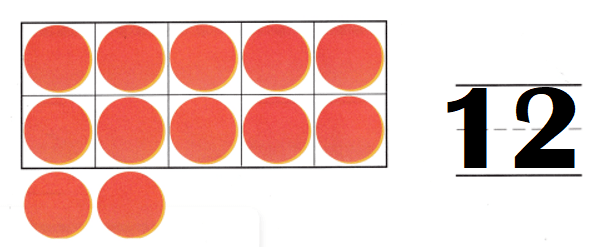Explanation:
Number of counters = 10 + 2 = 12.

### Texas Go Math Kindergarten Lesson 7.2 Homework and Practice Answer Key

DIRECTIONS: 1-2. Count and tell how many. Practice writing the number.

Question 1.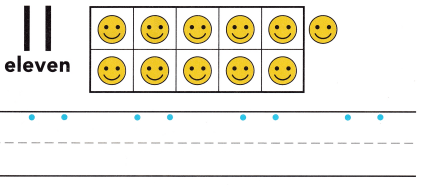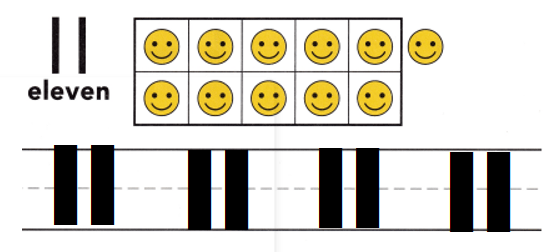Explanation:
Number of smileys = 11 or Eleven.

Question 2.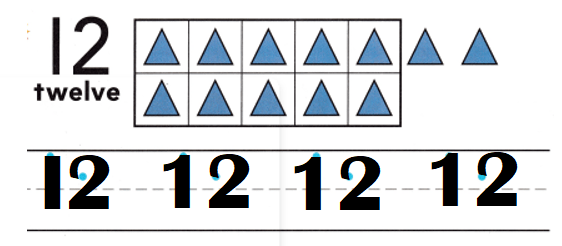Explanation:
Number of triangles = 12 or Twelve.

DIRECTIONS: Choose the correct answer. 3. Count the strawberries. How would you write the number? 4. Count the pears. How would you write the number? 5. Count the apples. How would you write the number?

Lesson Check
Question 3.Explanation:
number of strawberries = 11 or Eleven.

Question 4.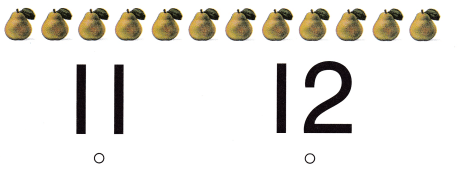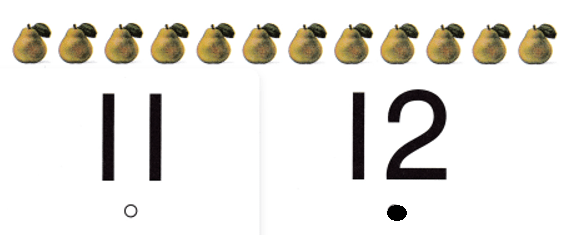Explanation:
Number of pears = 12 or Twelve.

Question 5.